# Simple interest And Compound interest for RRB PO & RRB Clerk – Quiz 8

## Simple interest And Compound interest for RRB PO & RRB Clerk – Quiz 8

Simple Interest and Compound Interest Questions form a majority of competitive examination papers. Simple Interest and Compound Interest Questions are a significant piece of the Quantitative Aptitude Section. So, here we present to you the Simple Interest and Compound Interest Quiz. This Simple Interest and Compound Interest Quiz is totally FREE for the Candidates. This Simple Interest and Compound Interest Quiz contains different kinds of questions ranging from simple to difficult level. This Simple Interest and Compound Interest Quiz will assist the competitors with upgrading their preparation for their upcoming exams like banking, Insurance, SSC, and Railway.

1. If Rs. 36000 is divided into two parts such that the simple interest on the first part for 3 years at 12% per annum is equal to the simple interest on the second part for 9/2 years at 16% per annum, then the larger part is
(a) Rs. 17000
(b) Rs. 18000
(c) Rs. 24000
(d) Rs. 12000
(e) Rs. 14000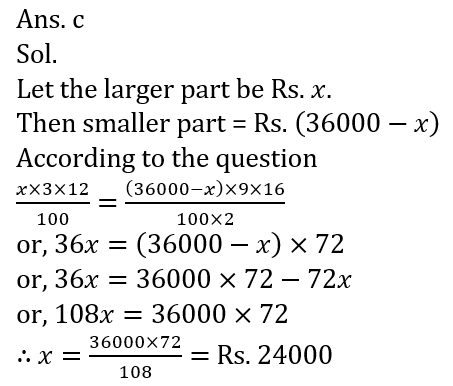2. Abhinav Invested X Rs. in SBI at the rate of 18% for 2 year and obtained a total simple interest of 6750 Rs. If he invested 2250 Rs. more for same period time at the rate of 100/7 % for first year and at 50/3 % for 2nd year on compound annually. Then find the total compound interest obtained by Abhinav after 2 year ?
(a) 6000 Rs.
(b) 5000 Rs.
(c) 3000 Rs.
(d) 7000 Rs.
(e) 5500 Rs.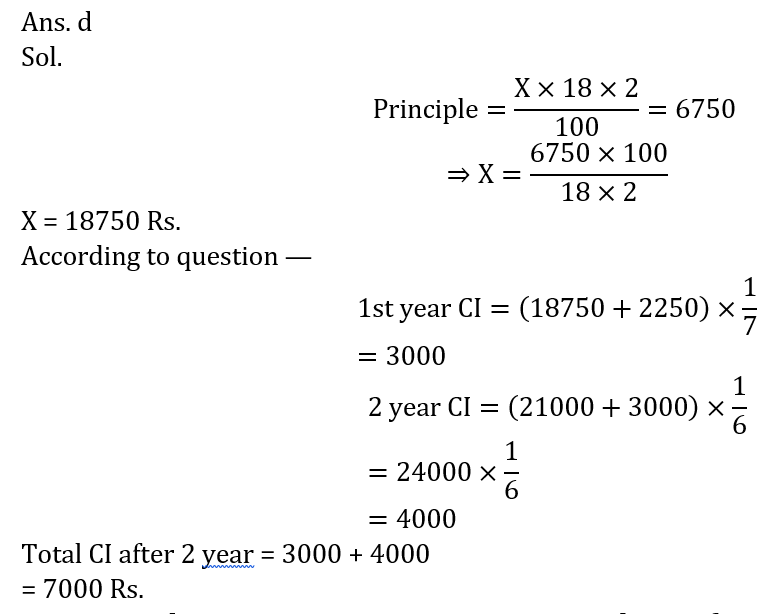3. Abhinav borrows Rs. 6000 at 30% compound rate of interest. At the end of each year he pays back Rs. 1200. How much amount should he pay at the end of the third year to clear all his dues?
(a) Rs. 9594
(b) Rs. 9584
(c) Rs. 9850
(d) Rs. 8574
(e) Rs. 89264. Same amounts are invested in two scheme with 8% interest for 2 years, one scheme at S.I. and another scheme at CI. If he received 41875.2 Rs. after 2 years then find the simple interest he earned.
(a) 3880
(b) 2000
(c) 2400
(d) 2500
(e) 2880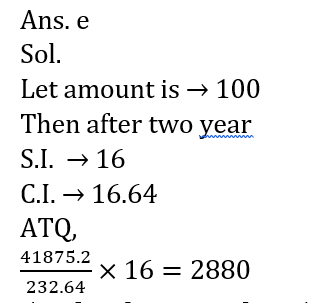5. Vikas borrowed Rs 50,000 from a bank at 20% per annum compound interest. At the end of every year, he paid Rs 15,000. If he wanted to clear his loan at the end of 3rd year, how much amount he needed to pay at the end of 3rd year ?
(a) Rs 32,680
(b) Rs 39,640
(c) Rs 48,760
(d) Rs 28,732
(e) Rs 46,8006. The interest received by Alpa by investing in a scheme which offers CI at 10% rate after two years is invested in a partnership with Abhishek. If ratio of Alpa and Abhishek’s investment in partnership is 3 : 4 and Alpa received Rs. 700 less profit than Abhishek, then find principle amount invested in CI scheme by Alpa ?
(a) Rs. 15000
(b) Rs. 20000
(c) Rs. 22000
(d) Rs. 25000
(e) cannot be determined

Ans. e
Sol.

7. Abhinav invested 40000 Rs. at the rate of 20% compound interest. If first year interest calculated half yearly and second year calculate annually, then find the total interest Veer gets after 2 year?
(a) 18080Rs.
(b) 19080 Rs.
(c) 18800Rs.
(d) 18600Rs.
(e) 18500Rs.8. A man invested Rs. 2250 at the rate of R% for 3/2 years on S.I. and got a total interest of Rs. 506.25. If he reinvested total amount and an additional amount of Rs. 43.75 at same interest rate on C.I. annually. Find total C.I. got by man at the end of two years ?
(a) Rs 1030
(b) Rs. 903
(c) Rs. 730
(d) Rs. 830
(e) Rs. 907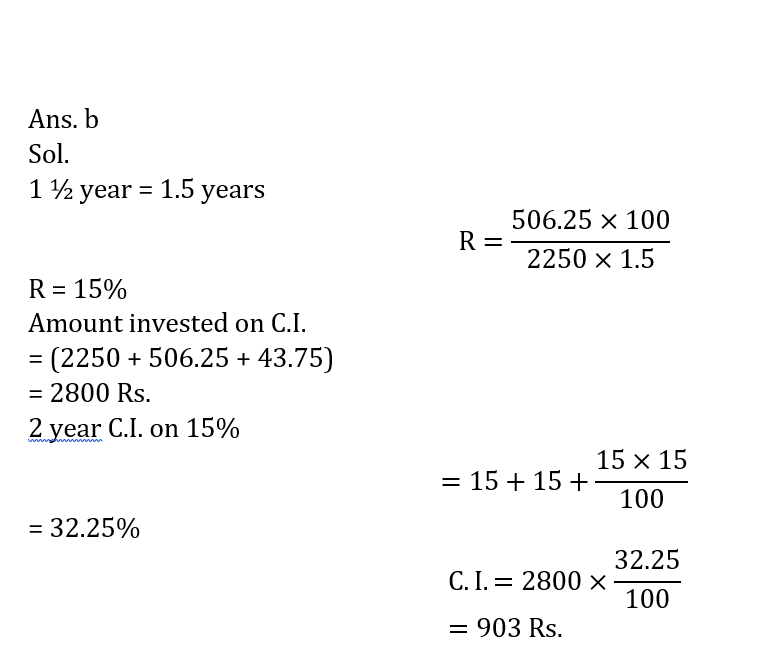9. X invested Rs. P at 10% per annum, Y invested Rs.(P – 4000) less amount than X at the rate of 12% per annum. If they both get total compound interest at the end of two years Rs. 6412.8, then find capital invested by Y ?
(a) 16000 Rs.
(b) 12000 Rs.
(c) 10000 Rs.
(d) 14000 Rs
(e) 18000 Rs.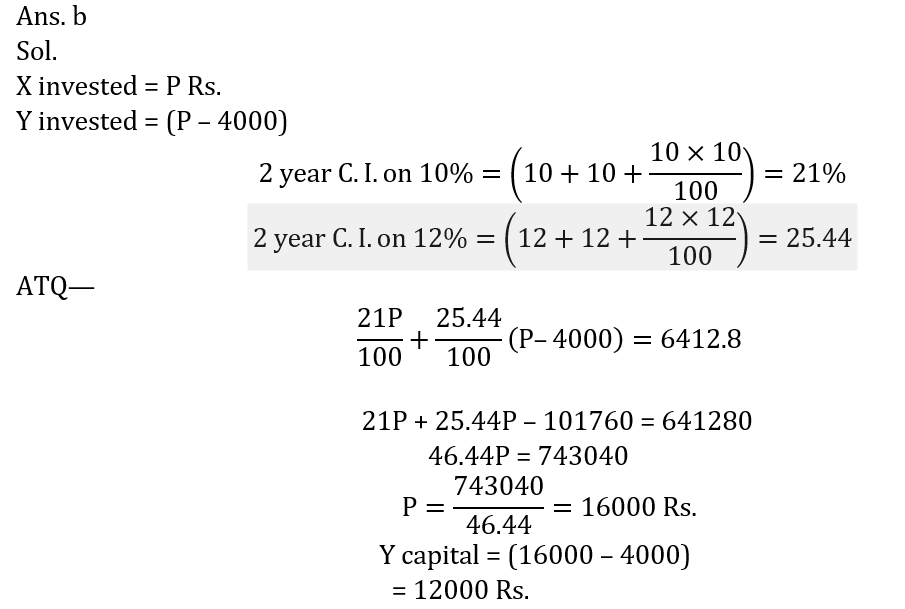10. A sum is invested at compound Interest at the rate of 8% per annum. If compound interest obtained only for second year is Rs. 4320. then find the amount invested. (in Rs.)
(a) 50000
(b) 45000
(c) 40000
(d) 52000
(e) 55000#### Attempt Quantitative Aptitude Topic Wise Online Test Series

Recommended PDF’s for :

#### Most important PDF’s for Bank, SSC, Railway and Other Government Exam : Download PDF Now

AATMA-NIRBHAR Series- Static GK/Awareness Practice Ebook PDF Get PDF here
The Banking Awareness 500 MCQs E-book| Bilingual (Hindi + English) Get PDF here
AATMA-NIRBHAR Series- Banking Awareness Practice Ebook PDF Get PDF here
Computer Awareness Capsule 2.O Get PDF here
AATMA-NIRBHAR Series Quantitative Aptitude Topic-Wise PDF Get PDF here
AATMA-NIRBHAR Series Reasoning Topic-Wise PDF Get PDF Here
Memory Based Puzzle E-book | 2016-19 Exams Covered Get PDF here
Caselet Data Interpretation 200 Questions Get PDF here
Puzzle & Seating Arrangement E-Book for BANK PO MAINS (Vol-1) Get PDF here
ARITHMETIC DATA INTERPRETATION 2.O E-book Get PDF here
3